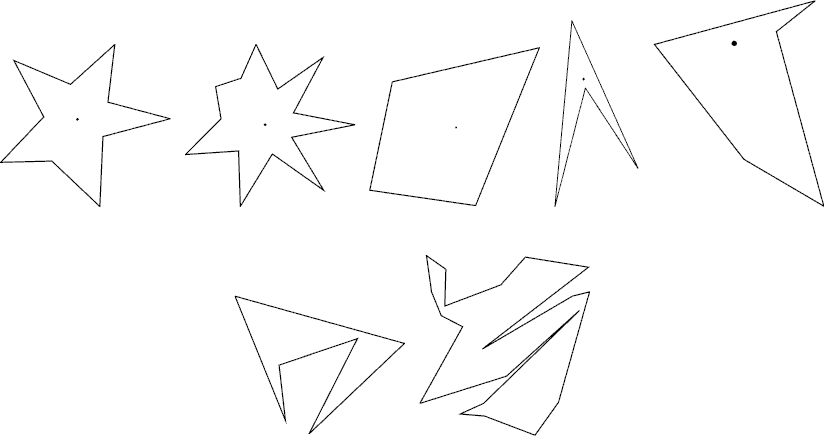• # POJ 3130 &amp; ZOJ 2820 How I Mathematician Wonder What You Are!（半平面相交 多边形是否有核）

Description

After counting so many stars in the sky in his childhood, Isaac, now an astronomer and a mathematician uses a big astronomical telescope and lets his image processing program count stars. The hardest part of the program is to judge if shining object in the sky is really a star. As a mathematician, the only way he knows is to apply a mathematical definition of stars.

The mathematical definition of a star shape is as follows: A planar shape F is star-shaped if and only if there is a point C ∈ F such that, for any point P ∈ F, the line segment CP is contained in F. Such a point C is called a center of F. To get accustomed to the definition let’s see some examples below.The first two are what you would normally call stars. According to the above definition, however, all shapes in the first row are star-shaped. The two in the second row are not. For each star shape, a center is indicated with a dot. Note that a star shape in general has infinitely many centers. Fore Example, for the third quadrangular shape, all points in it are centers.

Your job is to write a program that tells whether a given polygonal shape is star-shaped or not.

Input

The input is a sequence of datasets followed by a line containing a single zero. Each dataset specifies a polygon, and is formatted as follows.

 n x1 y1 x2 y2 … xn yn

The first line is the number of vertices, n, which satisfies 4 ≤ n ≤ 50. Subsequent n lines are the x- and y-coordinates of the n vertices. They are integers and satisfy 0 ≤ xi ≤ 10000 and 0 ≤ yi ≤ 10000 (i = 1, …, n). Line segments (xiyi)–(xi + 1yi + 1) (i = 1, …, n − 1) and the line segment (xnyn)–(x1y1) form the border of the polygon in the counterclockwise order. That is, these line segments see the inside of the polygon in the left of their directions.

You may assume that the polygon is simple, that is, its border never crosses or touches itself. You may assume assume that no three edges of the polygon meet at a single point even when they are infinitely extended.

Output

For each dataset, output “`1`” if the polygon is star-shaped and “`0`” otherwise. Each number must be in a separate line and the line should not contain any other characters.

Sample Input

```6
66 13
96 61
76 98
13 94
4 0
45 68
8
27 21
55 14
93 12
56 95
15 48
38 46
51 65
64 31
0```

Sample Output

```1
0```

Source

题意：

逆时针给出坐标。问此多边形是否有核！

代码例如以下：

```#include <cstdio>
#include <cmath>
#include <iostream>
using namespace std;
#define eps 1e-8
const int MAXN=10017;
int n;
double r;
int cCnt,curCnt;//此时cCnt为终于分割得到的多边形的顶点数、暂存顶点个数
struct point
{
double x,y;
};
point points[MAXN],p[MAXN],q[MAXN];//读入的多边形的顶点（顺时针）、p为存放终于分割得到的多边形顶点的数组、暂存核的顶点

void getline(point x,point y,double &a,double &b,double   &c){//两点x、y确定一条直线a、b、c为其系数
a = y.y - x.y;
b = x.x - y.x;
c = y.x * x.y - x.x * y.y;
}
void initial()
{
for(int i = 1; i <= n; i++)p[i] = points[i];
p[n+1] = p;
p = p[n];
cCnt = n;//cCnt为终于分割得到的多边形的顶点数。将其初始化为多边形的顶点的个数
}
point intersect(point x,point y,double a,double b,double c){//求x、y形成的直线与已知直线a、b、c、的交点
double u = fabs(a * x.x + b * x.y + c);
double v = fabs(a * y.x + b * y.y + c);
point pt;
pt.x=(x.x * v + y.x * u) / (u + v);
pt.y=(x.y * v + y.y * u) / (u + v);
return  pt;
}
void cut(double a,double b ,double c)
{
curCnt = 0;
int i;
for(i = 1; i <= cCnt; ++i)
{
if(a*p[i].x + b*p[i].y + c >= 0)q[++curCnt] = p[i];// c因为精度问题，可能会偏小，所以有些点本应在右側而没在，
//故应该接着推断
else
{
if(a*p[i-1].x + b*p[i-1].y + c > 0){//假设p[i-1]在直线的右側的话，
//则将p[i],p[i-1]形成的直线与已知直线的交点作为核的一个顶点(这种话，因为精度的问题，核的面积可能会有所降低)
q[++curCnt] = intersect(p[i],p[i-1],a,b,c);
}
if(a*p[i+1].x + b*p[i+1].y + c > 0){//原理同上
q[++curCnt] = intersect(p[i],p[i+1],a,b,c);
}
}
}
for(i = 1; i <= curCnt; ++i)p[i] = q[i];//将q中暂存的核的顶点转移到p中
p[curCnt+1] = q;p = p[curCnt];
cCnt = curCnt;
}
void solve()
{
//注意：默认点是顺时针，假设题目不是顺时针，规整化方向
initial();
for(int i = 1; i <= n; ++i)
{
double a,b,c;
getline(points[i],points[i+1],a,b,c);
cut(a,b,c);
}
/*
假设要向内推进r。用该部分取代上个函数
for(int i = 1; i <= n; ++i){
Point ta, tb, tt;
tt.x = points[i+1].y - points[i].y;
tt.y = points[i].x - points[i+1].x;
double k = r / sqrt(tt.x * tt.x + tt.y * tt.y);
tt.x = tt.x * k;
tt.y = tt.y * k;
ta.x = points[i].x + tt.x;
ta.y = points[i].y + tt.y;
tb.x = points[i+1].x + tt.x;
tb.y = points[i+1].y + tt.y;
double a,b,c;
getline(ta,tb,a,b,c);
cut(a,b,c);
}*/
/*   //多边形核的面积
double area = 0;
for(int i = 1; i <= curCnt; ++i)
area += p[i].x * p[i + 1].y - p[i + 1].x * p[i].y;
area = fabs(area / 2.0);
*/
}
/*void GuiZhengHua(){
//规整化方向，逆时针变顺时针。顺时针变逆时针
for(int i = 1; i < (n+1)/2; i ++)
swap(points[i], points[n-i]);
}*/
int main()
{
while(scanf("%d",&n) && n)
{
int i;
for(i = n; i >= 1; i--)//逆时针，顺时针反一下1->n就好了
{
scanf("%lf%lf",&points[i].x,&points[i].y);
}
points[n+1] = points;
solve();
if(cCnt < 1)
printf("0
");//无核
else
printf("1
");//有核
}
return 0;
}
```

• 相关阅读:
background之你不知道的background-position
ES6学习笔记（二）
ES6学习笔记（一）
将博客搬至CSDN
Mongodb的性能优化问题
使用AngularJS实现的前后端分离的数据交互过程
输出JS代码中的变量内容
程序生成word与PDF文档的方法(python)
python 2.7安装某些包出现错误："libxml/xmlversion.h：没有那个文件或目录"
Linux中安装配置spark集群
• 原文地址：https://www.cnblogs.com/wgwyanfs/p/7065578.html